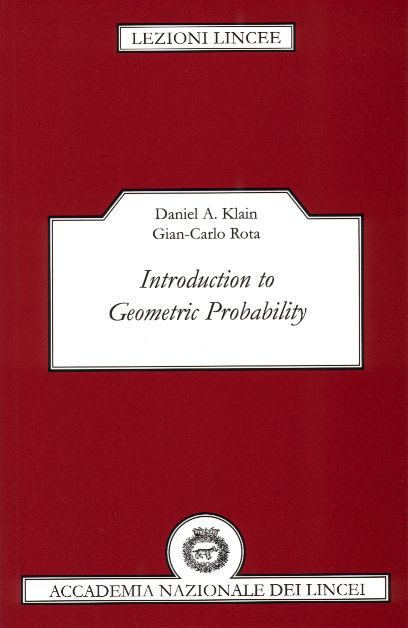## Introduction to Geometric Probability

by

Daniel A. Klain and Gian-Carlo Rota

This is a modern introduction to geometric probability, also known as integral geometry. The subject is presented at an elementary level, requiring little more than first year graduate mathematics. The theory of intrinsic volumes due to Hadwiger, McMullen, Santaló, and others is presented, along with a complete and elementary proof of Hadwiger's characterization theorem for invariant valuations in Euclidean n-space. The theory of the Euler characteristic is developed from an integral-geometric point of view. The authors then prove the fundamental theorem of integral geometry, namely the kinematic formula. Finally, the analogies between invariant valuations on polyconvex sets and valuations on order ideals of finite partially ordered sets are investigated. The relationship between convex geometry and enumerative combinatorics motivates much of the presentation. Every chapter concludes with a list of unsolved problems. Geometers and combinatorialists will find this a stimulating and fruitful tale.The book can be ordered through Amazon.com or your favorite local bookstore. You can also contact the publisher, Cambridge University Press.